## Erdös-Kac Theorem

A deeper result than the Hardy-Ramanujan Theorem. Let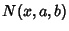be the number of Integers in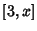such that inequality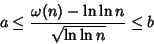holds, where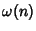is the number of different Prime factors of. Then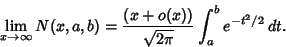The theorem is discussed in Kac (1959).

References

Kac, M. Statistical Independence in Probability, Analysis and Number Theory. New York: Wiley, 1959.

Riesel, H. The Erdös-Kac Theorem.'' Prime Numbers and Computer Methods for Factorization, 2nd ed. Boston, MA: Birkhäuser, pp. 158-159, 1994.